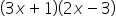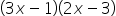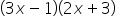Mathematics
Easy

Question

# Factorize the trinomial 6x2 + 7x – 3 as (px + q)(sx + t), where p, q, s and t are integers?

##Hint:

## The correct answer is:### Now we know that an algebraic expression known as a trinomial has three non-zero terms and more than one variable. An example of a trinomial is a polynomial having three terms. It is in the form of ax2+bx+c, for example: 5x4 - 4x2 +1.Here we have given the term as 6x2 + 7x – 3.We have:a = 6b = 7c = -3Now we will use middle term splitting method to factorise this expression, we get:

Here we used the concept of algebriac equations, trinomials and middle term splitting to factories the given expression. An expression with variables, constants, and algebraic operations is known as an algebraic expression (like subtraction, addition, multiplication, etc.). Terms comprise expressions. So the factorisation of 6x2 + 7x – 3 is (3x - 1)(2x + 3).

### Related Questions to study#### With Turito Foundation.#### Get an Expert Advice From Turito.